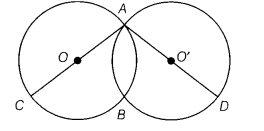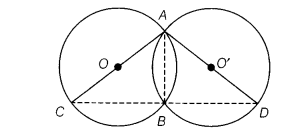# In the given figure, two circles intersect at A, B and AC, AD are respectively the diameters

In the given figure, two circles intersect at A, B and AC, AD are respectively the diameters of the circles. Prove that the points C, B and D are collinear.

Given Two circles with AC and AD as diameters respectively intersecting each other at A and B.

To prove Points C, B and D are collinear. Construction Join CB, BD and AB.We know that the angle in a semi-circle is 90°.
∠ABC = 90° and ∠ABD = 90°
[∴ AC and AD are diameters of circles]
Then, ∠ABC + ∠ABD = 90° + 90° = 180°
∴CBD is a straight line.
Hence, points C, B and D are collinear.